# Frank Solutions for Class 10 Maths Chapter 24 Measure of Central Tendency

Frank Solutions for Class 10 Maths Chapter 24 Measure of Central Tendency are useful for students as it helps them in scoring high marks in the examination. These solutions are prepared by subject matter experts at BYJU’S, describing the complete method of solving problems. Learners can download the pdf of Frank Solutions for Class 10 Maths Chapter 24 from the below links. It also provides the foundation for higher studies.

Chapter 24 – Measure of Central Tendency, is a single value that attempts to describe a set of data by identifying the central position within that set of data. Here we calculate the Frequency Distribution by using Frequency table, presentation of data in groups result in Grouping of Data. In the Frank Solutions, many such exercise problems are given which helps in better learning.

## Download the PDF of Frank Solutions for Class 10 Maths Chapter 24 Measure of Central Tendency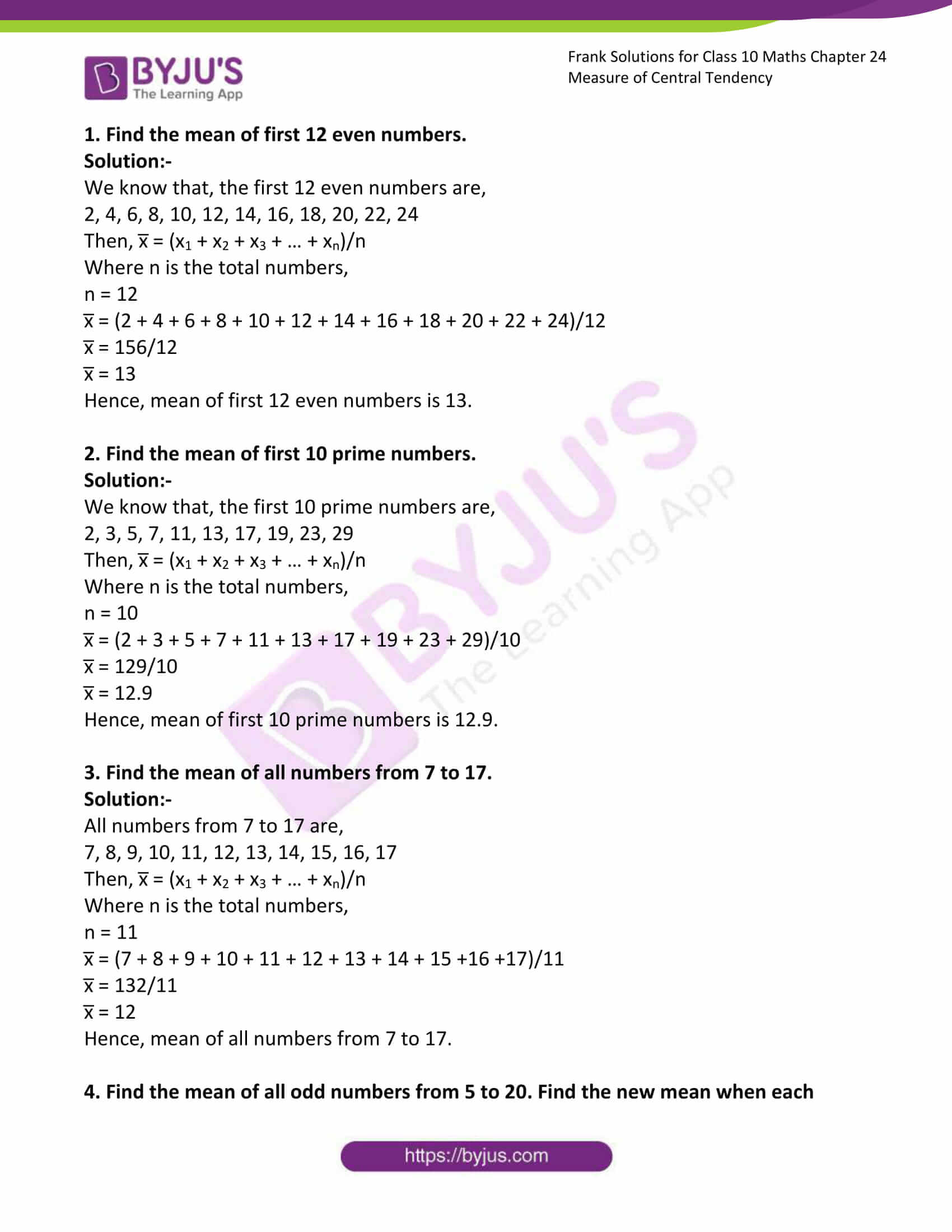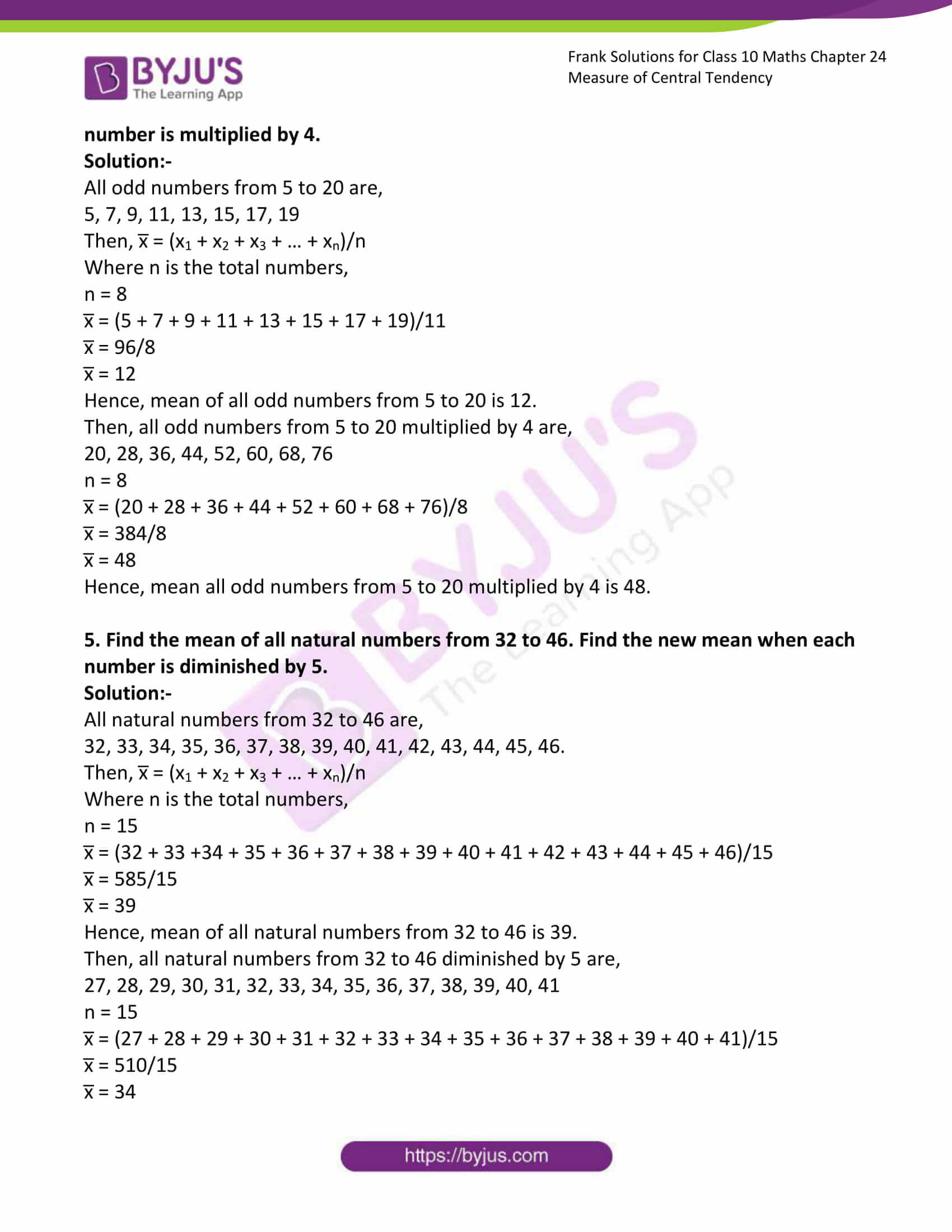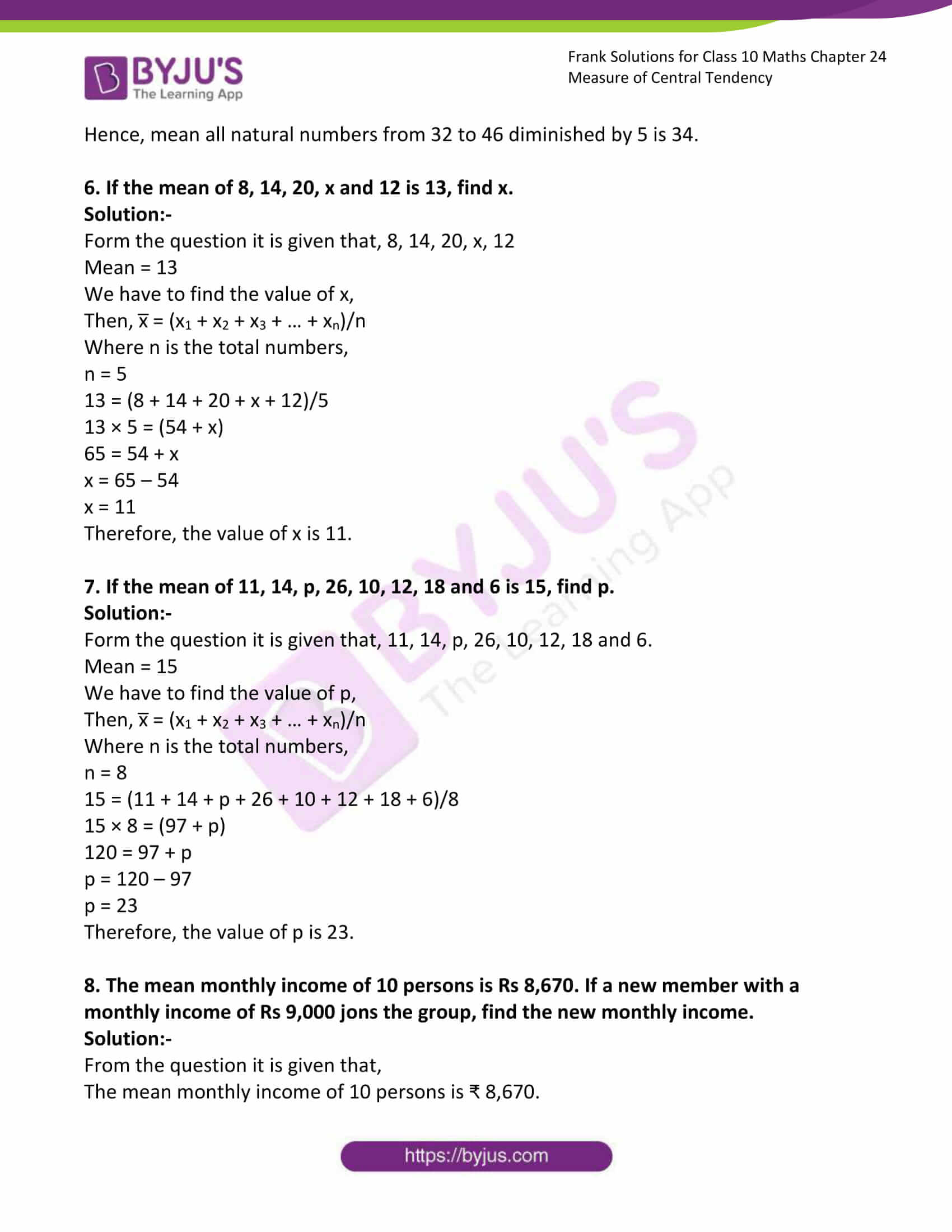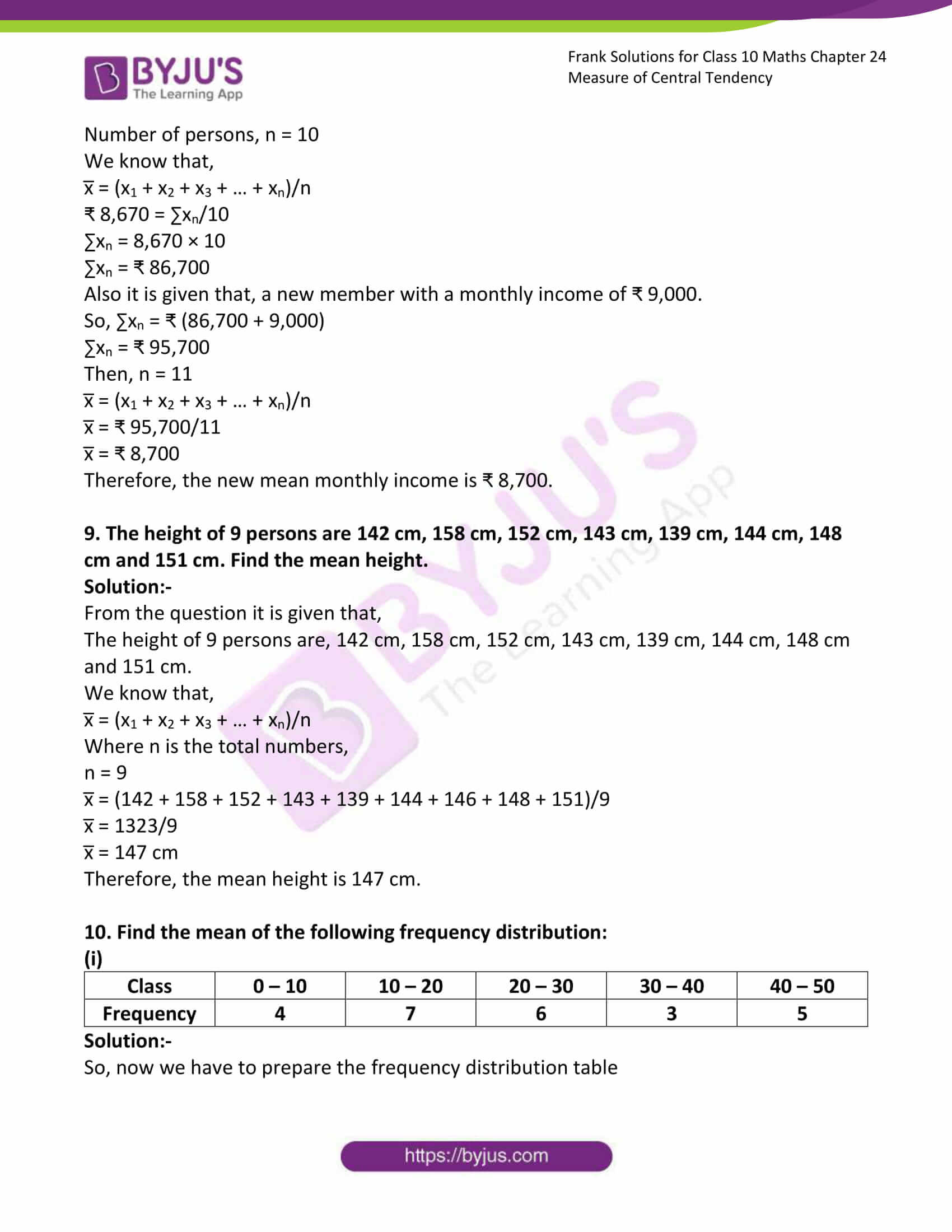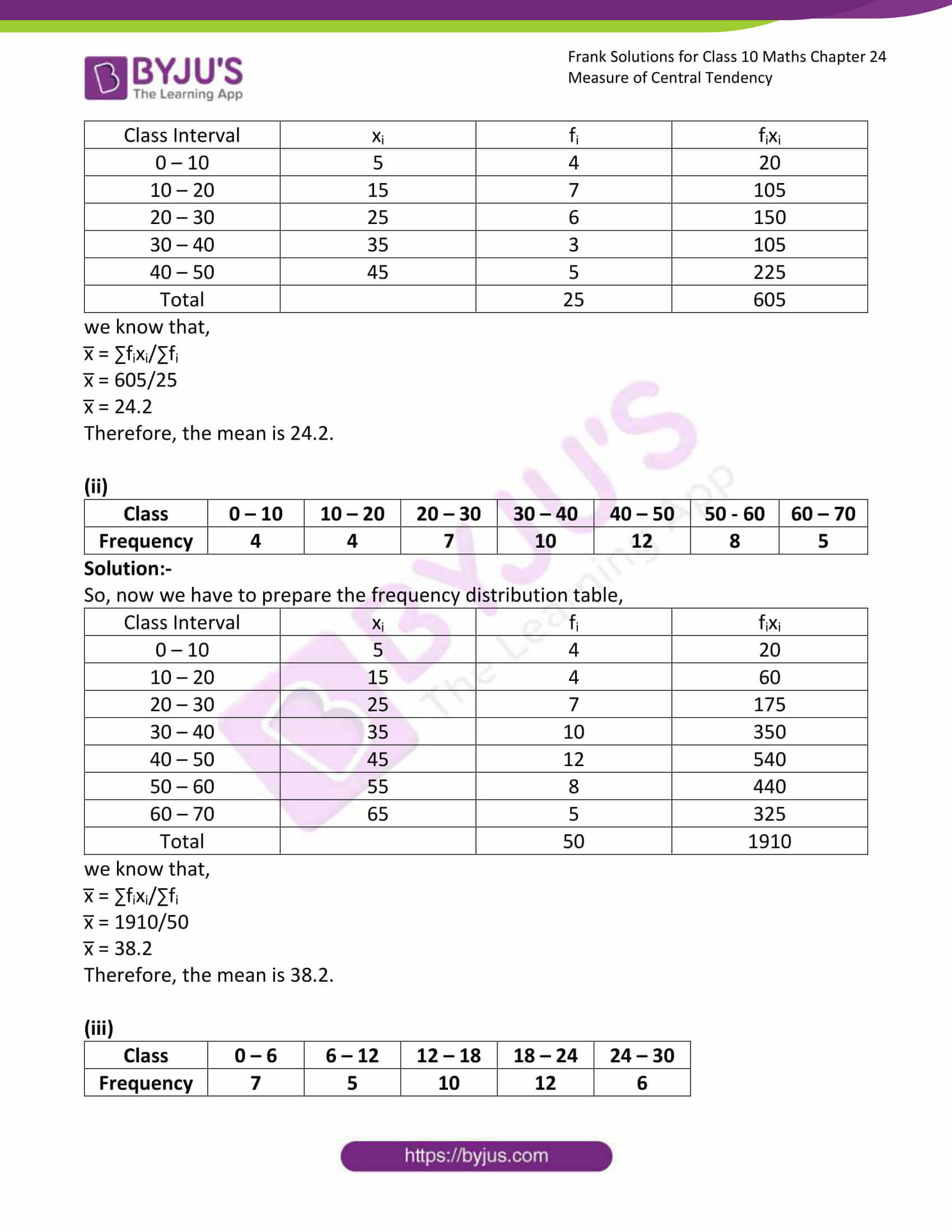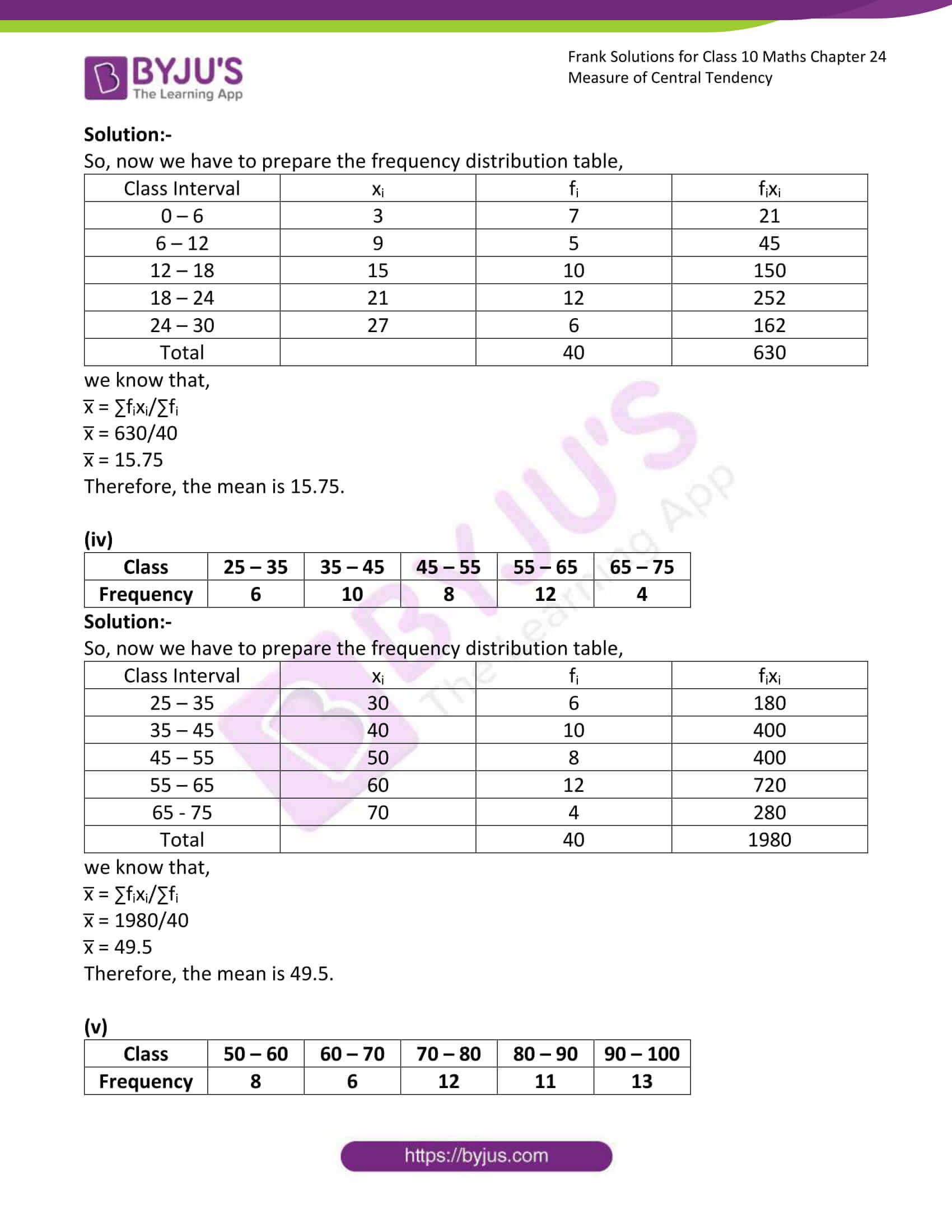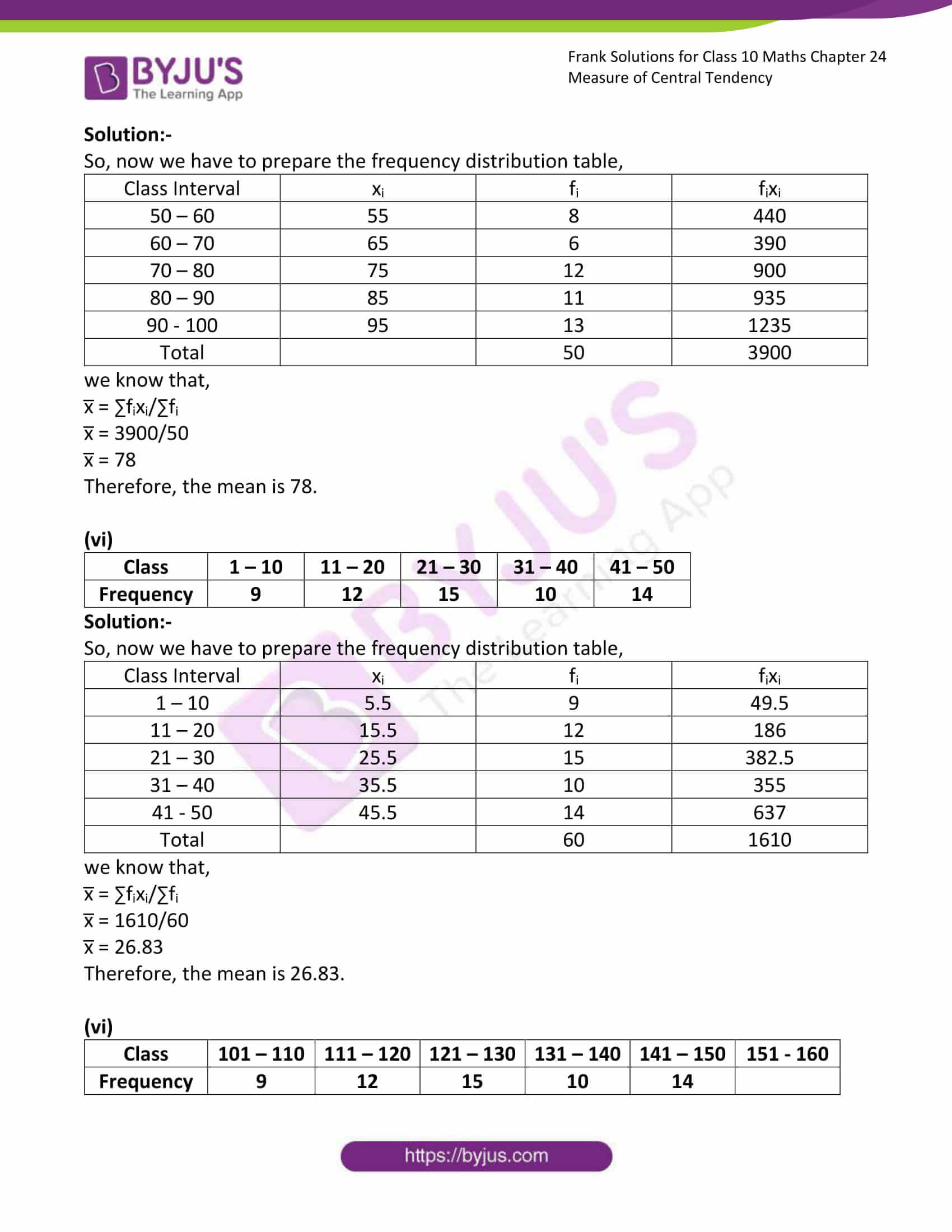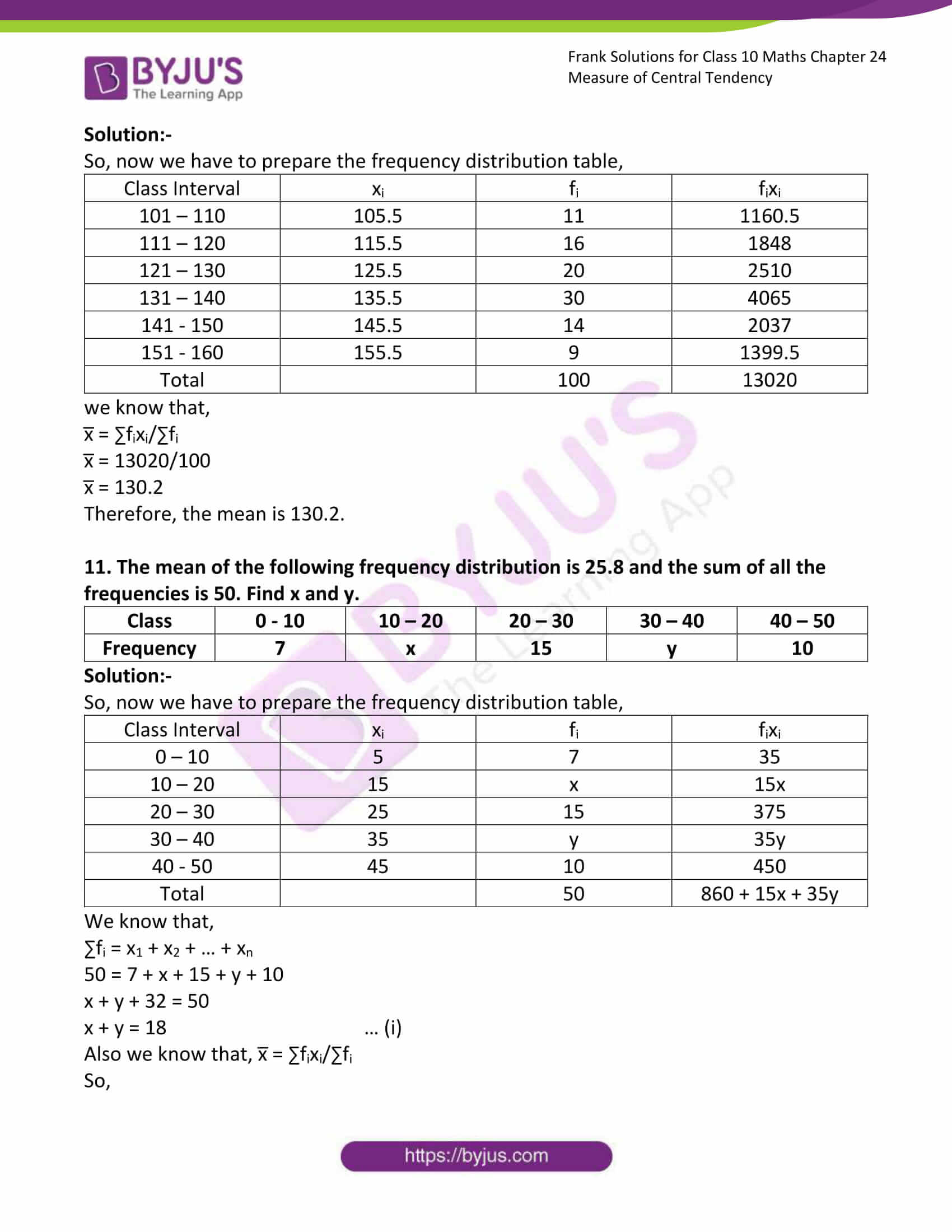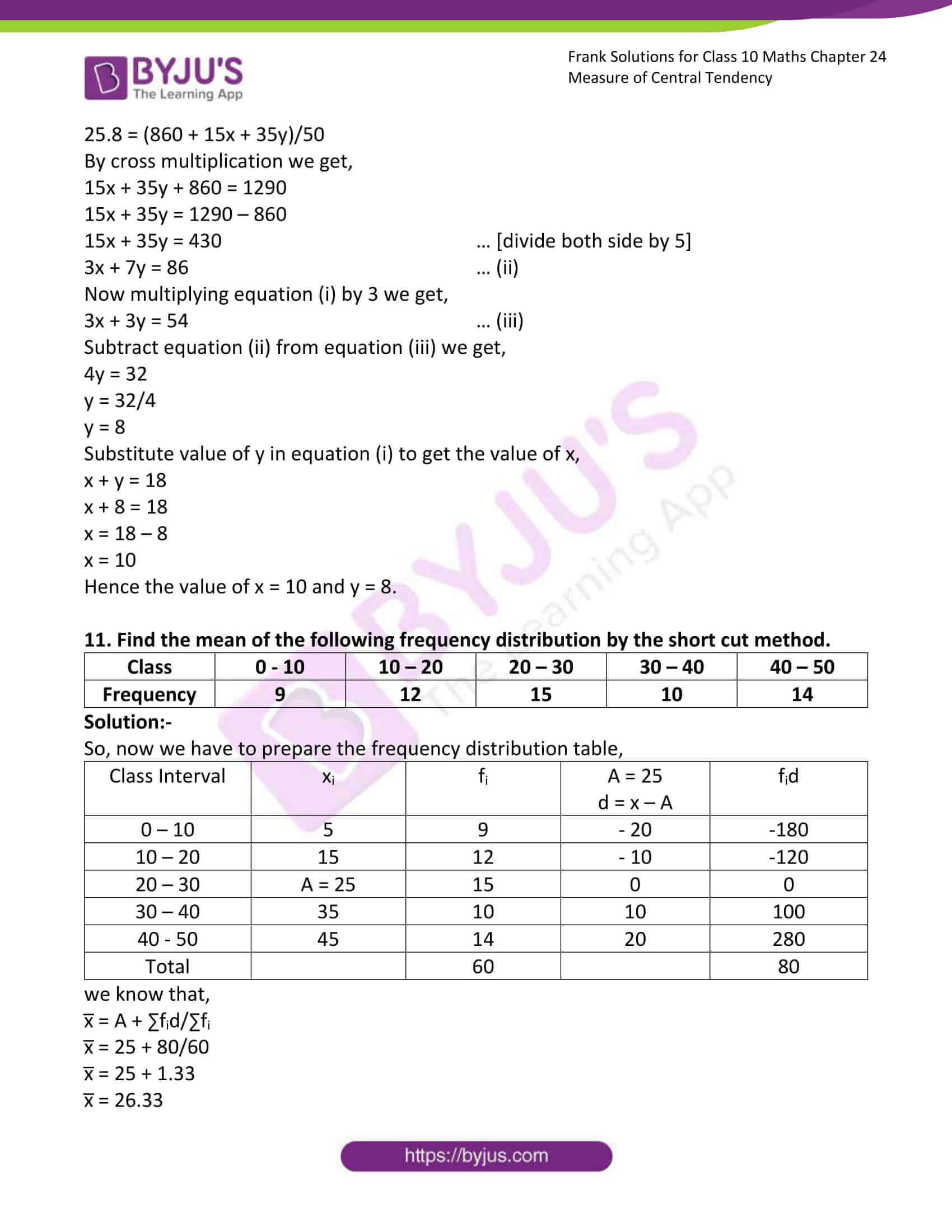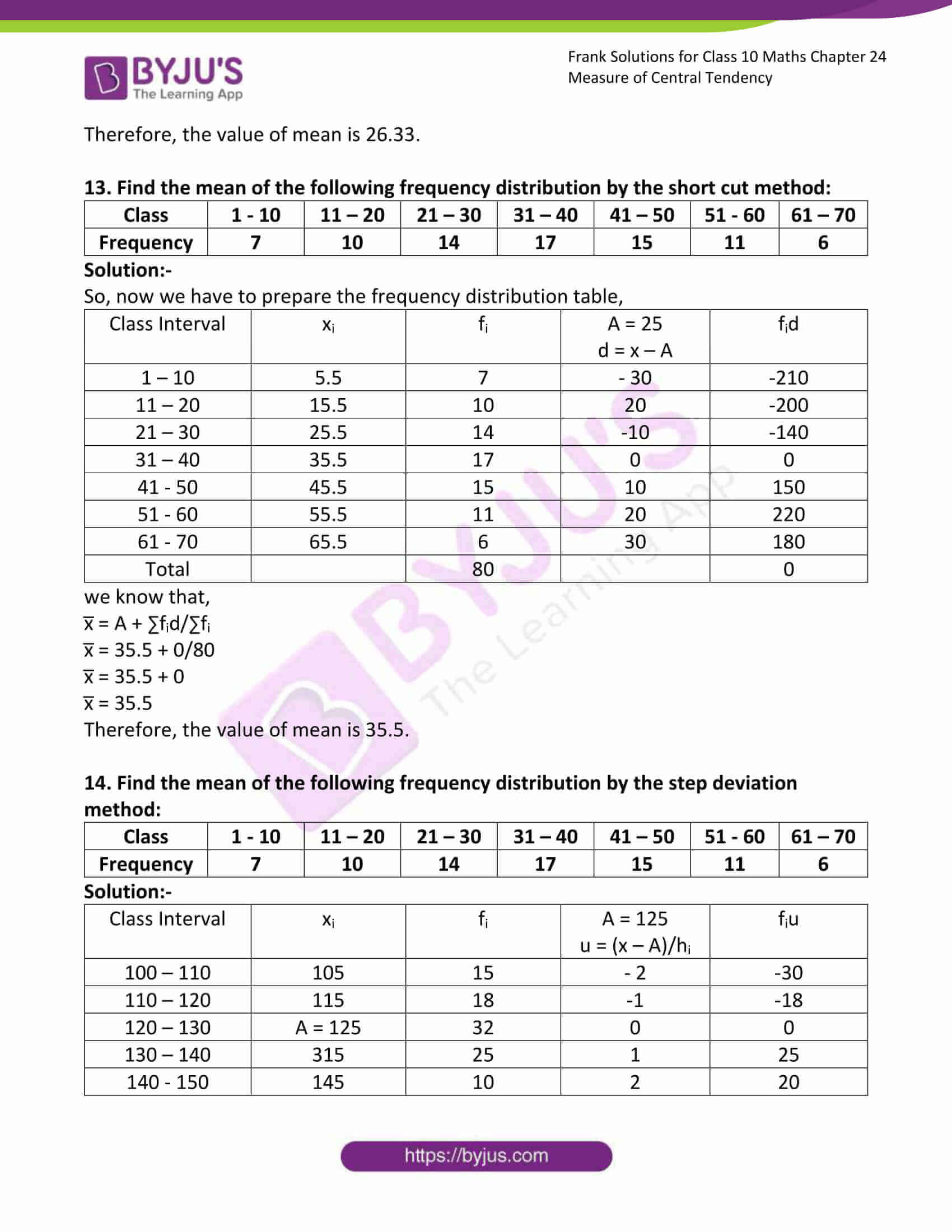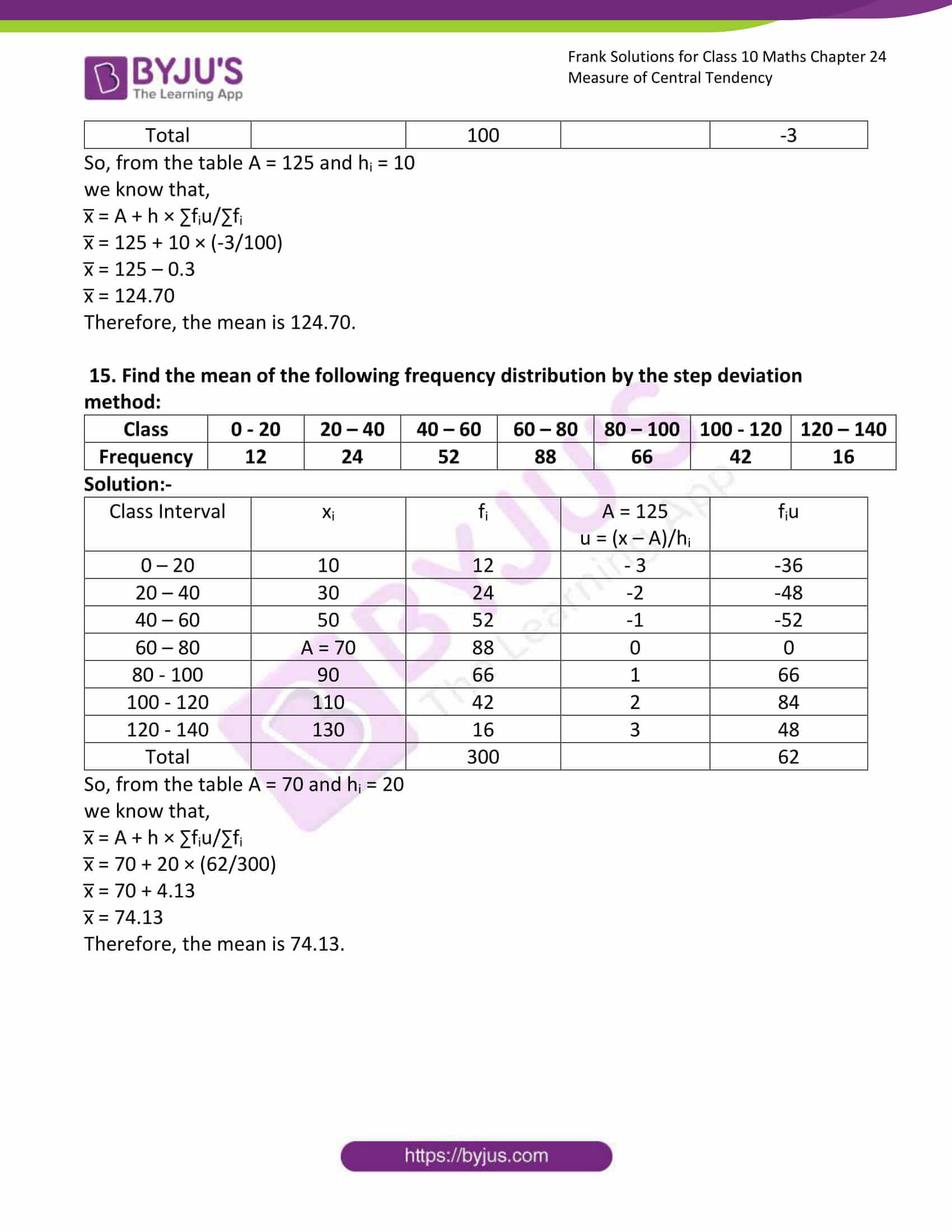### Access answers to Frank Solutions for Class 10 Maths Chapter 24 Measure of Central Tendency

1. Find the mean of first 12 even numbers.

Solution:-

We know that, the first 12 even numbers are,

2, 4, 6, 8, 10, 12, 14, 16, 18, 20, 22, 24

Then, x̅ = (x1 + x2 + x3 + … + xn)/n

Where n is the total numbers,

n = 12

x̅ = (2 + 4 + 6 + 8 + 10 + 12 + 14 + 16 + 18 + 20 + 22 + 24)/12

x̅ = 156/12

x̅ = 13

Hence, mean of first 12 even numbers is 13.

2. Find the mean of first 10 prime numbers.

Solution:-

We know that, the first 10 prime numbers are,

2, 3, 5, 7, 11, 13, 17, 19, 23, 29

Then, x̅ = (x1 + x2 + x3 + … + xn)/n

Where n is the total numbers,

n = 10

x̅ = (2 + 3 + 5 + 7 + 11 + 13 + 17 + 19 + 23 + 29)/10

x̅ = 129/10

x̅ = 12.9

Hence, mean of first 10 prime numbers is 12.9.

3. Find the mean of all numbers from 7 to 17.

Solution:-

All numbers from 7 to 17 are,

7, 8, 9, 10, 11, 12, 13, 14, 15, 16, 17

Then, x̅ = (x1 + x2 + x3 + … + xn)/n

Where n is the total numbers,

n = 11

x̅ = (7 + 8 + 9 + 10 + 11 + 12 + 13 + 14 + 15 +16 +17)/11

x̅ = 132/11

x̅ = 12

Hence, mean of all numbers from 7 to 17.

4. Find the mean of all odd numbers from 5 to 20. Find the new mean when each number is multiplied by 4.

Solution:-

All odd numbers from 5 to 20 are,

5, 7, 9, 11, 13, 15, 17, 19

Then, x̅ = (x1 + x2 + x3 + … + xn)/n

Where n is the total numbers,

n = 8

x̅ = (5 + 7 + 9 + 11 + 13 + 15 + 17 + 19)/11

x̅ = 96/8

x̅ = 12

Hence, mean of all odd numbers from 5 to 20 is 12.

Then, all odd numbers from 5 to 20 multiplied by 4 are,

20, 28, 36, 44, 52, 60, 68, 76

n = 8

x̅ = (20 + 28 + 36 + 44 + 52 + 60 + 68 + 76)/8

x̅ = 384/8

x̅ = 48

Hence, mean all odd numbers from 5 to 20 multiplied by 4 is 48.

5. Find the mean of all natural numbers from 32 to 46. Find the new mean when each number is diminished by 5.

Solution:-

All natural numbers from 32 to 46 are,

32, 33, 34, 35, 36, 37, 38, 39, 40, 41, 42, 43, 44, 45, 46.

Then, x̅ = (x1 + x2 + x3 + … + xn)/n

Where n is the total numbers,

n = 15

x̅ = (32 + 33 +34 + 35 + 36 + 37 + 38 + 39 + 40 + 41 + 42 + 43 + 44 + 45 + 46)/15

x̅ = 585/15

x̅ = 39

Hence, mean of all natural numbers from 32 to 46 is 39.

Then, all natural numbers from 32 to 46 diminished by 5 are,

27, 28, 29, 30, 31, 32, 33, 34, 35, 36, 37, 38, 39, 40, 41

n = 15

x̅ = (27 + 28 + 29 + 30 + 31 + 32 + 33 + 34 + 35 + 36 + 37 + 38 + 39 + 40 + 41)/15

x̅ = 510/15

x̅ = 34

Hence, mean all natural numbers from 32 to 46 diminished by 5 is 34.

6. If the mean of 8, 14, 20, x and 12 is 13, find x.

Solution:-

Form the question it is given that, 8, 14, 20, x, 12

Mean = 13

We have to find the value of x,

Then, x̅ = (x1 + x2 + x3 + … + xn)/n

Where n is the total numbers,

n = 5

13 = (8 + 14 + 20 + x + 12)/5

13 × 5 = (54 + x)

65 = 54 + x

x = 65 – 54

x = 11

Therefore, the value of x is 11.

7. If the mean of 11, 14, p, 26, 10, 12, 18 and 6 is 15, find p.

Solution:-

Form the question it is given that, 11, 14, p, 26, 10, 12, 18 and 6.

Mean = 15

We have to find the value of p,

Then, x̅ = (x1 + x2 + x3 + … + xn)/n

Where n is the total numbers,

n = 8

15 = (11 + 14 + p + 26 + 10 + 12 + 18 + 6)/8

15 × 8 = (97 + p)

120 = 97 + p

p = 120 – 97

p = 23

Therefore, the value of p is 23.

8. The mean monthly income of 10 persons is Rs 8,670. If a new member with a monthly income of Rs 9,000 jons the group, find the new monthly income.

Solution:-

From the question it is given that,

The mean monthly income of 10 persons is ₹ 8,670.

Number of persons, n = 10

We know that,

x̅ = (x1 + x2 + x3 + … + xn)/n

8,670 = ∑xn/10

∑xn = 8,670 × 10

∑xn = ₹ 86,700

Also it is given that, a new member with a monthly income of ₹ 9,000.

So, ∑xn = ₹ (86,700 + 9,000)

∑xn = ₹ 95,700

Then, n = 11

x̅ = (x1 + x2 + x3 + … + xn)/n

x̅ = ₹ 95,700/11

x̅ = ₹ 8,700

Therefore, the new mean monthly income is ₹ 8,700.

9. The height of 9 persons are 142 cm, 158 cm, 152 cm, 143 cm, 139 cm, 144 cm, 148 cm and 151 cm. Find the mean height.

Solution:-

From the question it is given that,

The height of 9 persons are, 142 cm, 158 cm, 152 cm, 143 cm, 139 cm, 144 cm, 148 cm and 151 cm.

We know that,

x̅ = (x1 + x2 + x3 + … + xn)/n

Where n is the total numbers,

n = 9

x̅ = (142 + 158 + 152 + 143 + 139 + 144 + 146 + 148 + 151)/9

x̅ = 1323/9

x̅ = 147 cm

Therefore, the mean height is 147 cm.

10. Find the mean of the following frequency distribution:

(i)

 Class 0 – 10 10 – 20 20 – 30 30 – 40 40 – 50 Frequency 4 7 6 3 5

Solution:-

So, now we have to prepare the frequency distribution table

 Class Interval xi fi fixi 0 – 10 5 4 20 10 – 20 15 7 105 20 – 30 25 6 150 30 – 40 35 3 105 40 – 50 45 5 225 Total 25 605

we know that,

x̅ = ∑fixi/∑fi

x̅ = 605/25

x̅ = 24.2

Therefore, the mean is 24.2.

(ii)

 Class 0 – 10 10 – 20 20 – 30 30 – 40 40 – 50 50 – 60 60 – 70 Frequency 4 4 7 10 12 8 5

Solution:-

So, now we have to prepare the frequency distribution table,

 Class Interval xi fi fixi 0 – 10 5 4 20 10 – 20 15 4 60 20 – 30 25 7 175 30 – 40 35 10 350 40 – 50 45 12 540 50 – 60 55 8 440 60 – 70 65 5 325 Total 50 1910

we know that,

x̅ = ∑fixi/∑fi

x̅ = 1910/50

x̅ = 38.2

Therefore, the mean is 38.2.

(iii)

 Class 0 – 6 6 – 12 12 – 18 18 – 24 24 – 30 Frequency 7 5 10 12 6

Solution:-

So, now we have to prepare the frequency distribution table,

 Class Interval xi fi fixi 0 – 6 3 7 21 6 – 12 9 5 45 12 – 18 15 10 150 18 – 24 21 12 252 24 – 30 27 6 162 Total 40 630

we know that,

x̅ = ∑fixi/∑fi

x̅ = 630/40

x̅ = 15.75

Therefore, the mean is 15.75.

(iv)

 Class 25 – 35 35 – 45 45 – 55 55 – 65 65 – 75 Frequency 6 10 8 12 4

Solution:-

So, now we have to prepare the frequency distribution table,

 Class Interval xi fi fixi 25 – 35 30 6 180 35 – 45 40 10 400 45 – 55 50 8 400 55 – 65 60 12 720 65 – 75 70 4 280 Total 40 1980

we know that,

x̅ = ∑fixi/∑fi

x̅ = 1980/40

x̅ = 49.5

Therefore, the mean is 49.5.

(v)

 Class 50 – 60 60 – 70 70 – 80 80 – 90 90 – 100 Frequency 8 6 12 11 13

Solution:-

So, now we have to prepare the frequency distribution table,

 Class Interval xi fi fixi 50 – 60 55 8 440 60 – 70 65 6 390 70 – 80 75 12 900 80 – 90 85 11 935 90 – 100 95 13 1235 Total 50 3900

we know that,

x̅ = ∑fixi/∑fi

x̅ = 3900/50

x̅ = 78

Therefore, the mean is 78.

(vi)

 Class 1 – 10 11 – 20 21 – 30 31 – 40 41 – 50 Frequency 9 12 15 10 14

Solution:-

So, now we have to prepare the frequency distribution table,

 Class Interval xi fi fixi 1 – 10 5.5 9 49.5 11 – 20 15.5 12 186 21 – 30 25.5 15 382.5 31 – 40 35.5 10 355 41 – 50 45.5 14 637 Total 60 1610

we know that,

x̅ = ∑fixi/∑fi

x̅ = 1610/60

x̅ = 26.83

Therefore, the mean is 26.83.

(vi)

 Class 101 – 110 111 – 120 121 – 130 131 – 140 141 – 150 151 – 160 Frequency 9 12 15 10 14

Solution:-

So, now we have to prepare the frequency distribution table,

 Class Interval xi fi fixi 101 – 110 105.5 11 1160.5 111 – 120 115.5 16 1848 121 – 130 125.5 20 2510 131 – 140 135.5 30 4065 141 – 150 145.5 14 2037 151 – 160 155.5 9 1399.5 Total 100 13020

we know that,

x̅ = ∑fixi/∑fi

x̅ = 13020/100

x̅ = 130.2

Therefore, the mean is 130.2.

11. The mean of the following frequency distribution is 25.8 and the sum of all the frequencies is 50. Find x and y.

 Class 0 – 10 10 – 20 20 – 30 30 – 40 40 – 50 Frequency 7 x 15 y 10

Solution:-

So, now we have to prepare the frequency distribution table,

 Class Interval xi fi fixi 0 – 10 5 7 35 10 – 20 15 x 15x 20 – 30 25 15 375 30 – 40 35 y 35y 40 – 50 45 10 450 Total 50 860 + 15x + 35y

We know that,

∑fi = x1 + x2 + … + xn

50 = 7 + x + 15 + y + 10

x + y + 32 = 50

x + y = 18 … (i)

Also we know that, x̅ = ∑fixi/∑fi

So,

25.8 = (860 + 15x + 35y)/50

By cross multiplication we get,

15x + 35y + 860 = 1290

15x + 35y = 1290 – 860

15x + 35y = 430 … [divide both side by 5]

3x + 7y = 86 … (ii)

Now multiplying equation (i) by 3 we get,

3x + 3y = 54 … (iii)

Subtract equation (ii) from equation (iii) we get,

4y = 32

y = 32/4

y = 8

Substitute value of y in equation (i) to get the value of x,

x + y = 18

x + 8 = 18

x = 18 – 8

x = 10

Hence the value of x = 10 and y = 8.

11. Find the mean of the following frequency distribution by the short cut method.

 Class 0 – 10 10 – 20 20 – 30 30 – 40 40 – 50 Frequency 9 12 15 10 14

Solution:-

So, now we have to prepare the frequency distribution table,

 Class Interval xi fi A = 25 d = x – A fid 0 – 10 5 9 – 20 -180 10 – 20 15 12 – 10 -120 20 – 30 A = 25 15 0 0 30 – 40 35 10 10 100 40 – 50 45 14 20 280 Total 60 80

we know that,

x̅ = A + ∑fid/∑fi

x̅ = 25 + 80/60

x̅ = 25 + 1.33

x̅ = 26.33

Therefore, the value of mean is 26.33.

13. Find the mean of the following frequency distribution by the short cut method:

 Class 1 – 10 11 – 20 21 – 30 31 – 40 41 – 50 51 – 60 61 – 70 Frequency 7 10 14 17 15 11 6

Solution:-

So, now we have to prepare the frequency distribution table,

 Class Interval xi fi A = 25 d = x – A fid 1 – 10 5.5 7 – 30 -210 11 – 20 15.5 10 20 -200 21 – 30 25.5 14 -10 -140 31 – 40 35.5 17 0 0 41 – 50 45.5 15 10 150 51 – 60 55.5 11 20 220 61 – 70 65.5 6 30 180 Total 80 0

we know that,

x̅ = A + ∑fid/∑fi

x̅ = 35.5 + 0/80

x̅ = 35.5 + 0

x̅ = 35.5

Therefore, the value of mean is 35.5.

14. Find the mean of the following frequency distribution by the step deviation method:

 Class 1 – 10 11 – 20 21 – 30 31 – 40 41 – 50 51 – 60 61 – 70 Frequency 7 10 14 17 15 11 6

Solution:-

 Class Interval xi fi A = 125 u = (x – A)/hi fiu 100 – 110 105 15 – 2 -30 110 – 120 115 18 -1 -18 120 – 130 A = 125 32 0 0 130 – 140 315 25 1 25 140 – 150 145 10 2 20 Total 100 -3

So, from the table A = 125 and hi = 10

we know that,

x̅ = A + h × ∑fiu/∑fi

x̅ = 125 + 10 × (-3/100)

x̅ = 125 – 0.3

x̅ = 124.70

Therefore, the mean is 124.70.

15. Find the mean of the following frequency distribution by the step deviation method:

 Class 0 – 20 20 – 40 40 – 60 60 – 80 80 – 100 100 – 120 120 – 140 Frequency 12 24 52 88 66 42 16

Solution:-

 Class Interval xi fi A = 125 u = (x – A)/hi fiu 0 – 20 10 12 – 3 -36 20 – 40 30 24 -2 -48 40 – 60 50 52 -1 -52 60 – 80 A = 70 88 0 0 80 – 100 90 66 1 66 100 – 120 110 42 2 84 120 – 140 130 16 3 48 Total 300 62

So, from the table A = 70 and hi = 20

we know that,

x̅ = A + h × ∑fiu/∑fi

x̅ = 70 + 20 × (62/300)

x̅ = 70 + 4.13

x̅ = 74.13

Therefore, the mean is 74.13.